# A System Approach to Understanding the Impact of Nonideal Effects in a Motor Drive Current Loop

An essential part of any digitally controlled motor drive is phase current feedback. The quality of the measurement directly relates to system parameters such as torque ripple and torque settling time. While there is strong correlation between system performance and phase current measurement, it is difficult to translate into hard requirements to the feedback system. From a system point of view, this article discusses how to design a feedback system optimized for motor control. Error sources are identified and mitigation effects are discussed.

### 1. Introduction

The performance of the current loop in a motor drive or servo (see Figure 1) directly impacts the torque output from the motor, which is critical for a smooth response, as well as accurate positioning and speed profiles. A key measure of smooth torque output is torque ripple. This is particularly important for profiling and cutting applications where torque ripple directly translates into achievable end application precision. Parameters related to the current loop dynamics, such as response time and settling time, are very important for automation applications where production efficiency is directly impacted by the available control bandwidth. Apart from the motor design itself, multiple factors within the drive directly impact these performance parameters.Figure 1. Current loop in a motor drive with nonideal elements in the feedback path.

There are several sources of torque ripple within a motor drive. Some are from the motor itself, such as cogging torque due to stator winding and slot arrangement and rotor EMF harmonics.1 Other sources of torque ripple are related to offset and gain error in the phase current feedback system2 (see Figure 1).

Inverter dead time also directly impacts torque ripple in that it adds low frequency (predominantly 5th and 7th)3 harmonic components of the stator electrical frequency to the PWM output voltage. The impact on the current loop in this case is related to the disturbance rejection of the current loop at the harmonic frequencies.

This article will focus on torque ripple due to phase current measurement. Each of the errors are analyzed and approaches to minimizing the effect of measurement error are discussed.

### 2. Torque Ripple Due to Current Measurement Error

The electromagnetic torque equation of a 3-phase permanent magnet motor is:Te is the electromagnetic torque, PP is the number of pole pairs, λPM is the permanent magnet flux, Ld and Lq are the stator inductances in the synchronous rotating reference frame, and id and iq are the stator currents in the synchronous rotating reference frame. In steady state and under ideal conditions, id and iq are dc quantities and consequently, the produced torque will also be a dc quantity. A torque ripple will be present when there is an ac component in id or iq. Because of the direct relationship between idq and the produced torque the approach used in this article is to analyze how various measurements errors affect id and iq. As a basis for this analysis, consider the current feedback for a 3-phase motor:Where ix is the measured phase current (x = a,b,c), ix1 is the actual phase current and ixe is the measurement error. No assumptions are made about the nature of the error; it can be an offset, a gain error, or an ac component. Using the Clarke transformation, the currents are projected onto stationary 2-phase quantities iα and iβ:Using the Park transformation, the currents are projected onto rotating 2-phase quantities id and iq:where θ is the angle of the rotor. For field oriented control of a 3-phase motor it is necessary to know all three phase currents. One common approach is to measure all three currents, which naturally requires three sensors and three feedback channels. Another common approach is to only measure two channels and calculate the third current. For cost and complexity reasons, it is desirable to have fewer sensors and measurement channels but as will be explained, measuring all three currents makes the system much more robust toward measurement errors.

#### 2.1 Two-Phase Measurement

First consider a 3-phase drive that has two phase current measurements. The third phase current is calculated with the assumption that the currents sum to 0. If ia and ib are measured, ic is calculated as: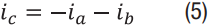Using equation (2) and (5):In the stationary reference frame, the currents are: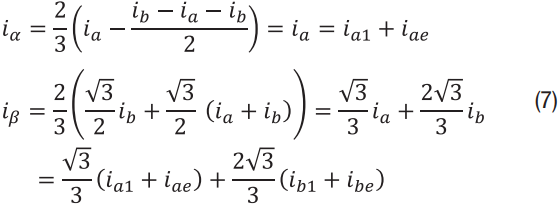In the rotating reference frame, the currents are:Notice how both id and iq have a term that relates to the true phase current and a term that relates to the measurement error (idq = idq1+ idqe). For this analysis the error terms ide and iqe are of most interest:#### 2.2 Three-Phase Measurement

Now consider a 3-phase drive that measures all three phase currents. Following the same procedure as with two channels, the stationary and rotating quantities are derived: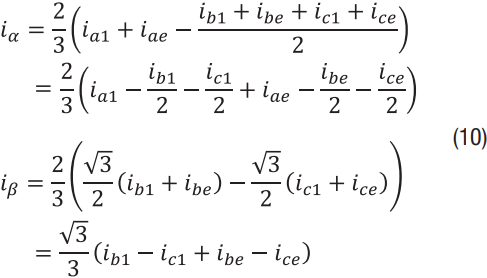And in rotating frame:Again the equations have a term that relates to the true phase current (idq1) and a term that relates to the measurement error (idqe). The error terms ide and iqe are:### 3. Incorrect Sampling Instant

When a 3-phase motor is fed by a switching voltage source inverter, the phase current can be seen as two components: a fundamental component and a switching component (see Figure 2A).Figure 2. (A) Phase currents of a 3-phase motor driven by a switching voltage source inverter. (B) Zoom of a phase current illustrating how the current ripple is attenuated through sampling.

For control purposes, the switching component has to be eliminated or it will affect the performance of the current control loop. A common technique to extract the average component is to sample the currents synchronized to the PWM cycle. At the beginning and at the center of a PWM cycle the currents assume their average value and if sampling is tightly synchronized to these instances the switching component is effectively suppressed, as shown in Figure 2B. However, if the current is sampled with a timing error, aliasing will occur and as a consequence performance of the current loop drops. This section discusses reasons for timing errors, what the effects on the current loop are, and finally how to make the system robust to sample timing errors.

#### 3.1 Sample Timing Errors in a Motor Drive

With the fundamental component of a phase current typically in the range of tens of Hz and the bandwidth of the current loop in the range of a few kHz, it seems counter intuitive that a small timing error can affect control performance. However, with only the phase inductance to limit di/dt, even a small timing error will result in significant current distortion. For example, 250 V across an inductor of 5 mH for 1 μs will change the current by 50 mA. In addition, assume the system uses a 12-bit ADC with a full scale of 10 A, then the lower 4.3 bits of the ADC are lost because of the timing error. As will be shown, losing bits is the best case scenario. The aliasing can also cause torque ripple as well as gain error in the feedback system.

The most common reasons for an incorrect sampling instant are:

• Insufficient link between the PWM and the ADC, making it impossible to sample at the right time.
• A lack of sufficient (two or three depending on the number of phases being measured) independent simultaneous sample-and-hold circuits.
• Propagation delay on gate drive signals which will bring the motor voltage out phase with the PWM timer.

Generally, anything that can impact di/dt determines how severe an incorrect sampling instant is. Of course the size of the timing error is important but system parameters such as motor speed, load, motor impedance, and dc-bus voltage also have a direct influence on the error.

#### 3.2 Effect of Sampling Error on System Performance

Using the derived equations, the effect of sampling error can be determined. With 2-phase current measurement, assume ia is sampled at the ideal instant (iae = 0) and ib is sampled with a delay, which results results in ibe ≠ 0. In this case the error terms defined by Equation 9 are:With 3-phase current measurement, assume ia and ic are sampled at the ideal instant (iae = ice = 0) and ib is sampled with a delay (ibe ≠ 0). In this case the error terms defined by Equation 12 are:From Equations 13 and 14 some interesting conclusions can be made. First of all, the Clarke/Park transformation gains the measurement error differently:So if the feedback system has a delay on one of the current measurements, the impact on a drive with two channels will be 1.73 times higher than if the system had three channels.

Using Equations 13 and 14, it is also possible to identify the impact of measurement delay on the motor torque. For this analysis it is assumed the phase current is sampled while applying a zero voltage to the motor terminals (V000 or V111) and during this period, the only voltage driving di/dt is the BEMF. With a sinusoidal BEMF, di/dt will also follow a sinusoidal function—that is di/dt = 0 at BEMF zero cross and di/dt at maximum when the BEMF peaks. Now, if a phase current is sampled with a fixed delay with respect to the ideal sampling instant the error is sinusoidal: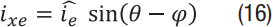Where x = a,b,c and φ is the phase angle with respect to the dq reference frame. Using ide from Equation 13 as an example:The term cos(– φ) is an offset while cos(2 θ – φ) is an ac component oscillating at twice the fundamental frequency. With these components in the dq currents, the motor torque will have similar components. Another thing to note is that with three current measurements, the chosen orientation of the dq frame φ = –π, which means the offset term is zero. That is, no gain error with three channels. The difference between a two and three sensor system is illustrated in Figure 3.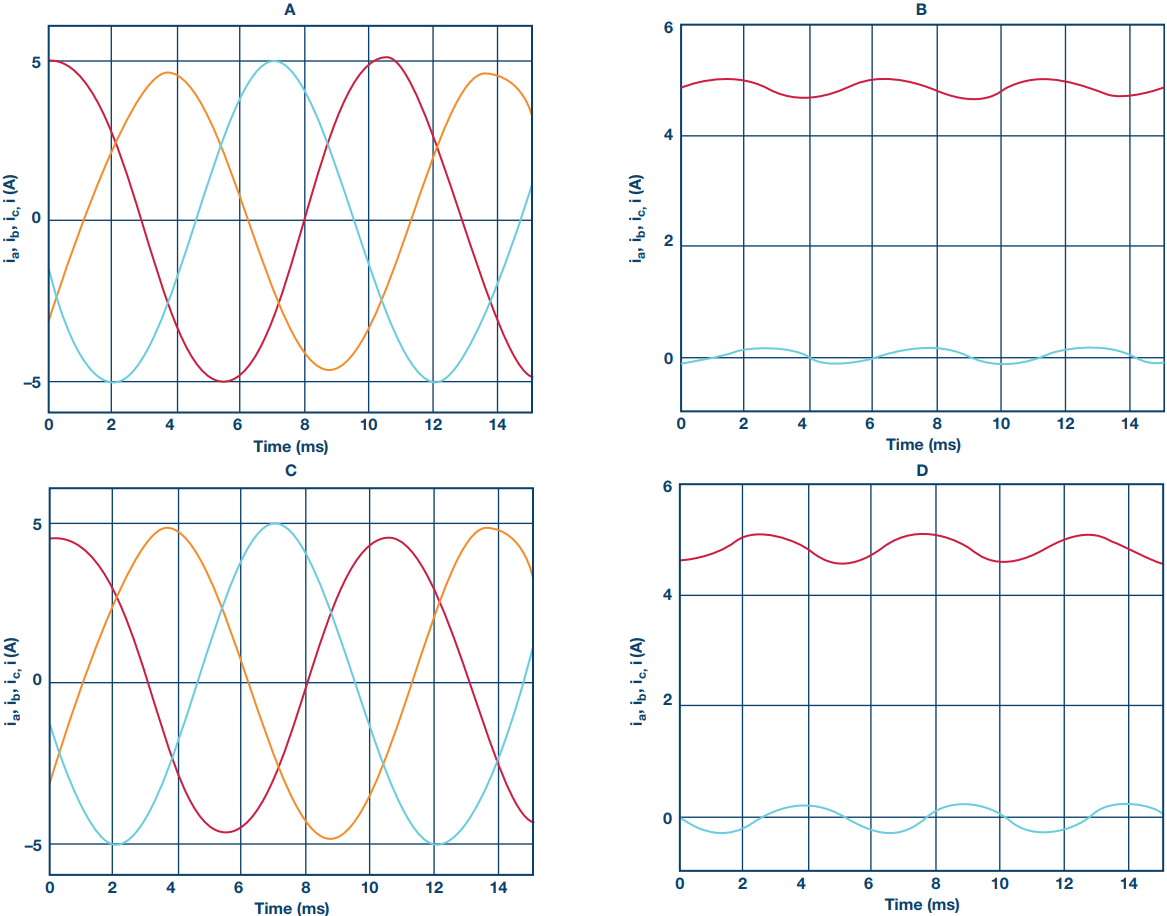Figure 3: Effect of an incorrect sampling instant. ia,ib,ic and id,iq with two current sensors (A, B) and three current sensors (C, D).

In the three sensor case from Figures 3A and 3B, notice how the delay on ib measurement results in a current (torque ripple) at 2 times the fundamental frequency. Also notice how the dc components of id and iq are not affected.

In the two sensor case, from Figures 3C and 3D, notice how the delay on ib measurement results in an ac component that is 1.73 times higher than with three sensors. In addition, the dc components of both id and iq are also affected.

#### 3.3 Minimizing the Effect Sample Timing Error

As the performance requirements of the control loop increases it becomes desirable to minimize the effect of sample timing errors, especially when bearing in mind the trend toward increased ADC resolution. A few years ago 10- to 12-bit ADCs were common but now a resolution of 16 bits is becoming the norm. These additional bits should be utilized—otherwise the value of a high performing ADC will be compromised with the lower bits lost due to delays in the system.

The most effective way to minimize the effect of sampling timing error is to get as close as possible to the ideal sampling instant for all phases. This may result in choosing a controller that is optimized for digital control switching power converters. In addition, optimizing propagation delay/skewing in the gate drive circuit will have a positive impact.

If timing error minimization still does not meet requirements, a significant performance improvement can be achieved by using three current sensors and an ADC with three independent sample-and-hold circuits.

### 4. Offset Error

The derived equations can also describe how the system reacts to an offset on the measured currents. First, by looking at the two sensor case and using ide from Equation 9 as an example, the error component can be expressed as: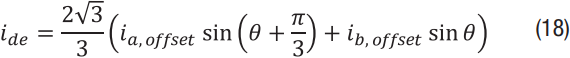With ia,offset and ib,offset the offset of the a and b channels, respectively. As can be seen, the offset will cause an ac component in the currents (and torque) at the fundamental frequency of the motor. If the system has offset calibration at startup, any remaining offset will be due to drift. In this case assuming the sensors drift in the same manner, the approximation ia,offset = ib,offset = ioffset can be made.This means the amplitude of the error component is twice the amplitude of the phase offset. A similar result can be found for the q-axis component of the error current. Performing the same exercise with three current sensors, it is found that ide from Equation 12 is:And following the reasoning that initial offset is calibrated out and all sensors drift equally, ia,offset = ib,offset = ic,offset = ioffset:Again, the benefit of having three sensors is clear—the offset on the current sensors will have no effect of torque ripple. Even if the sensors do not drift in exactly the same way they are likely to show the same trend. Therefore, a three sensor setup will always have significantly lower torque ripple in a system with uncalibrated offset errors.

#### 4.1 Minimizing the Effect of Offset Error

Offset on current feedback is one of the dominating sources of torque ripple in a motor drive and it is desirable to minimize it as much as possible. Generally speaking there are two types of offset errors on current feedback. Firstly, there is the static offset that is present at any point in time and at any temperature. Secondly, there is the offset drift that is a function of parameters such as temperature and time. A common technique to minimize the effect of static offset is to do offset calibration that can be done either at the time of manufacture or every time when the motor current is 0 (typically when the motor is stopped). If this approach is used, static offset is usually not a concern.

Offset drift is more complicated to handle. As this is a slow drift that usually happens while the motor is operating, it is difficult to do online calibration and stopping the motor is generally not an option. Some online calibration techniques based on observers have been suggested,4 but the observers rely on models of the motor’s electrical and mechanical system. For the online estimation to be effective, exact knowledge of the motor parameters is required and that is usually not the case.

As was previously discussed, the most effective mitigation of offset drift is to use three current measurements. Assuming the channels use the same type of components, the drift of the channels are likely to be similar. If this is the case, the offsets cancel out and will not result in a torque ripple. Even if the channels do not drift at the same rate, as long as they drift in the same direction, the three channel approach will have a canceling effect on the offset.

With two current measurements, the torque ripple is present even if the channels drift at the same rate. In other words, a two sensor system is very sensitive to offset drift. In this case the only way avoid torque ripple is to make sure the drift is kept low which may add cost and complexity to the feedback system. For a given set of performance requirements a 3-channel feedback system may prove to be the cost-effective solution.

### 5. Gain Error

When the system has a gain error on current feedback, the error signal, ixe, is proportional to the actual phase current, ix1 (x = a, b, c):This is a sinusoidal error at the fundamental frequency. As can be seen, the nature of an error due to gain is similar to an error due to incorrect sample timing, (see Equation 16). Therefore, the same conclusions can be drawn:

• If the same gain error is present on all channels, there will be no torque ripple; only a gain error. This applies to 2-channel and 3-channel systems.
• If the gain error varies from one channel to the other, it will result in a torque ripple component at twice the fundamental frequency.
• 2-channel current measurement is 1.73 times more sensitive to gain error than a 3-channel current measurement.

### 6. Experimental Validation

The effect of offset error and gain error on the measured current and output torque are validated in the experimental setup illustrated in Figure 4.

The current feedback circuit in the drive board utilizes Hall effect transducers in the three motor phases. 2-phase or 3-phase current measurement can be selected in the software. Offset calibration is performed when the motor is not running, so under normal operation (without allowing time for drift effects) offset and gain error are quite small. In order to illustrate the effects of these errors, which will typically be present due to temperature drift (in spite of calibration routines), artificial offset and gain errors are introduced in the control software after the calibration routine. The measured quantities as seen by the control algorithm will differ from the actual quantities, which will contain the effects of the errors as discussed in previous sections. Figure 5 illustrates this for a set speed reference of 520 rpm—hence a motor electrical frequency of 35 Hz.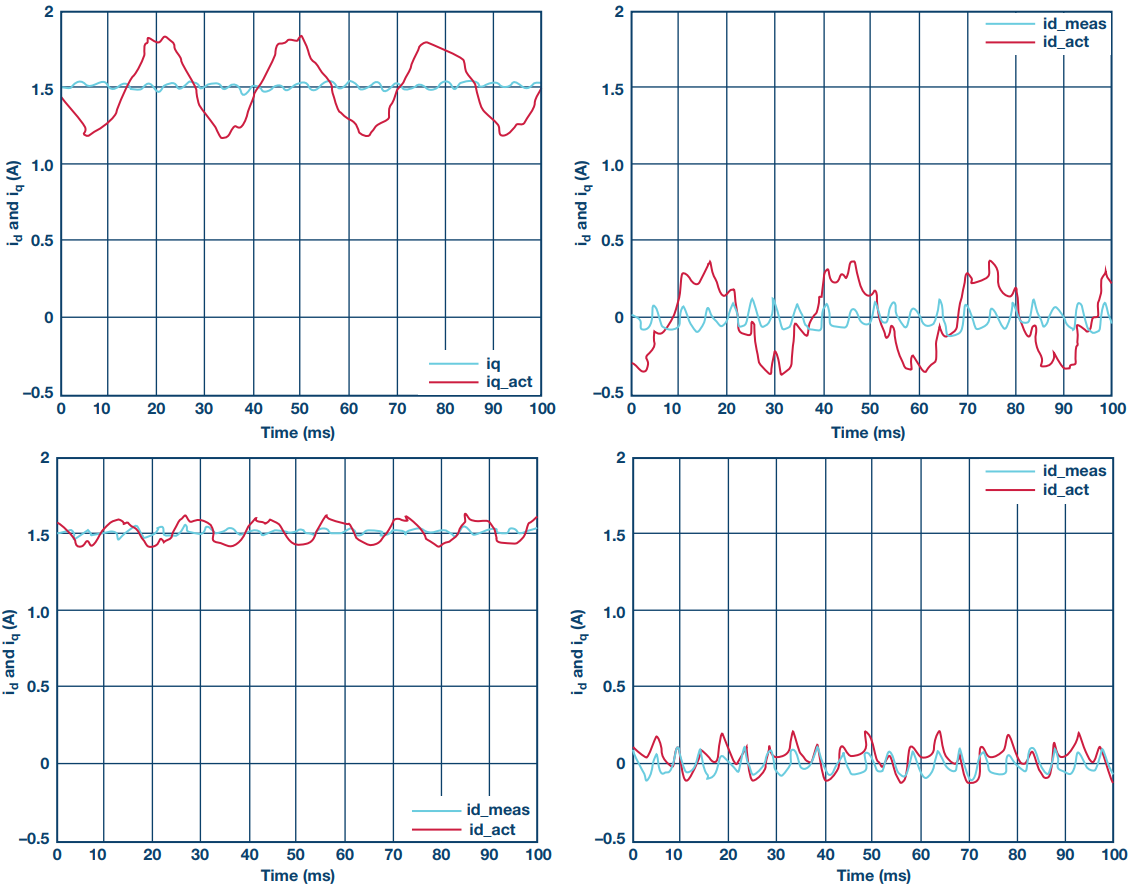Figure 5. Actual (red) and measured (blue) (top to bottom); iq and id with 1% offset error; iq and id with asymmetric gain error (1.05/0.95).

It is clear that while the drive is controlling the d-axis and q-axis currents to relatively constant values in order to maintain the set speed, the actual currents contain significant harmonic components, especially in the case of offset error. These harmonic components directly impact the output torque ripple. This is illustrated in Figure 6. It must be noted that there is a significant mechanical torque pulsation due to a slight shaft misalignment in the test rig. This is present at the mechanical frequency and some low order harmonics. However, the variation in harmonic content related to the offset and gain error sources is still clearly seen. For the offset error, the harmonic component at the electrical frequency (35 Hz) increases in proportion with the offset error percentage, as illustrated, while the harmonic content at twice the electrical frequency increases with the gain error asymmetry, as predicted by the theory.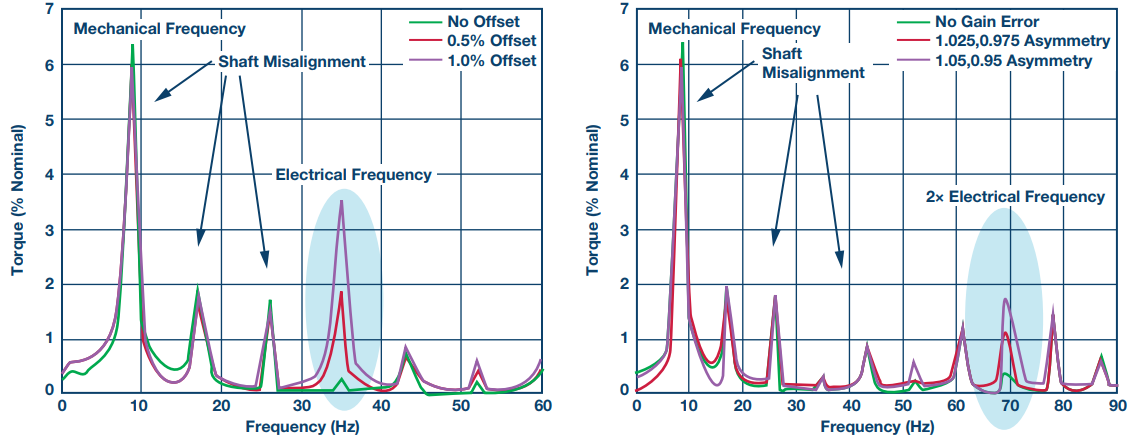Figure 6. Measured torque ripple as % of nominal with 2-phase current measurement and (left) increasing offset error and (right) increasing gain error.

Moreover, the impact of 3-phase measurement is clearly seen in Figure 7, where the offset error induced torque ripple is completely eliminated and the gain error-induced torque ripple is reduced by a factor of 1.73—again bearing out the theoretical calculations.Figure 7. Measured torque ripple as % of nominal with 3-phase current measurement and (left) increasing offset error and (right) increasing gain error.

### Summary

Through analysis and measurement, this article has shown how nonideal effects in the current feedback system affect system performance. It has been shown how a system with three current measurements is significantly more robust toward measurement error than a system with two current measurements.

### 参考資料

1 Weizhe Qian, Sanjib K. Panda, and Jian-Xin Xu. “Torque Ripple Minimization in PM Synchronous Motors Using Iterative Learning Control.” IEEE Transactions on Power Electronics, Vol. 19, No. 2, 2004.

2 Dae-Woong Chung, Seung-Ki Sul, and Dong-Choon Lee, “Analysis and Compensation of Current Measurement Error in Vector Controlled AC Motor Drives.” Industry Applications Conference, 1996. Thirty-First IAS Annual Meeting, IAS 1996., Conference Record of the 1996 IEEE, Vol.1, 1996.

3 Somyo Kaitwanidvilai, Werachet Khan-ngern, and Montri Panarut. “The Impact of Deadtime Effect on Unwanted Harmonics Conducted Emission of PWM Inverters.” Environmental Electromagnetics, 2000. CEEM 2000. Proceedings. Asia-Pacific Conference on, Shanghai, 2000.

4 Yutaro Uenaka, Masaki Sazawa, and Kiyoshi Ohishi, “Fine Self-Tuning Method of Both Current Sensor Offset and Electrical Parameter Variations for SPM Motor.” IECON 2010—36th Annual Conference on IEEE Industrial Electronics Society, 2010.